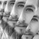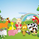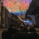2194 views
2194
This is an experimental study designed to filter out minor price action for a clearer view of trends.

Inspired by the QQE's volatility filter, this filter applies the process directly to price rather than to a smoothed RSI .

First, a smooth average price range is calculated for the basis of the filter and multiplied by a specified amount.
Next, the filter is calculated by gating price movements that do not exceed the specified range.
Lastly the target ranges are plotted to display the prices that will trigger filter movement.

Custom bar colors are included. The color scheme is based on the filtered price trend.sfigus
@sfigus, Use whatever timeframe you like. The default settings work with any resolution. The only thing that changes with timeframe is the width of the average range in the calculation, which you could increase or decrease manually using the range multiplier input.Hey, this is some great work and I'm really trying to make sense of everything happening here but I have a question.

rngfilt := x > nz(rngfilt) ? ((x - r) < nz(rngfilt) ? nz(rngfilt) : (x - r)) : ((x + r) > nz(rngfilt) ? nz(rngfilt) : (x + r))

I cannot understand the logic behind the ternary conditions above because it's the first time I've seen it as ? ? :

I could only find 2 ways to use ternary conditions. x = var 1 ? var2 : var 3 and x = cond1 ? x : cond2 ? y : cond3 ? z

In the first it's x = var2 if var1 = true, and var3 if var1 = false.
Whereas the second example is your barcolor variable.

But the rngfilt I cannot understand. Could you please explain the logic of the operations?

On a side-note, why is the "wper" variable named as such?

Thank you!